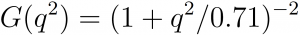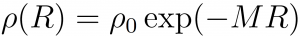# Funding Areas

FOR MORE RESEARCH TOPICS

## The Radial Density of the Proton’s Quarks

A handful of experiments provide information on the proton form factor. The data is described by the phenomenological “dipole formula” whose form (in GeV) is (see , pp. 196; , p. 175)(1)

When multiplying this formula with appropriate factors, one obtains the electric and the magnetic form factors. It means that this is the quarks form factor. An analogous expression holds for the neutron’s magnetic form factor (see , pp. 194-195).

An appropriate Fourier transformation yields the exponentially decaying form of the radial dependence of quark density (see , pp. 195-196; , p. 175)(2)

This formula of the proton’s radial dependence of quark density corresponds to the well-known radial dependence of the ground state of the electron in the hydrogen atom, which is determined by the laws of electrodynamics (see e.g. , p. 210).

Research topic #1: Find the quark dynamical laws yielding the above data.

Research topic #2: Is the above data consistent with QCD’s asymptotic freedom?

Research topic #3: Consider the following experimental information: conservation of parity in strong and electromagnetic interactions; the interaction of hard photons with hadrons; ordinary liquids and the nuclear liquid drop model; the similarity between the graphs of molecular force (see , p. 16) and that of the nuclear force (see , p. 97); the EMC effect; the similarity between the electron/quark radial dependence of density (2). Is there an inherent law that yields this amazing correspondence between strong interactions and electromagnetic interactions?

#### References

 D. H. Perkins, Introduction to High Energy Physics (Addison-Wesley, Menlo Park CA, 1987).

 M. Thomson, Modern Particle Physics (Cambridge University Press, Cambridge UK, 2013).

 E. Merzbacher, Quantum Mechanics (John Wiley, New York, 1970)

 H. Haken and H. C. Wolf, Molecular Physics and Elements of Quantum Chemistry (Springer, Berlin, 2004).

 S. S. M. Wong, Introductory Nuclear Physics (Wiley, New York, 1998).